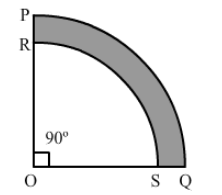# In the given figure, PQRS represents a flower bed.`
Question:

In the given figure, PQRS represents a flower bed. If OP = 21 m and OR = 14 m, find the area of the flower bed.Solution:

Area of the flower bed is the difference between the areas of sectors OPQ and ORS.

Area of the flower bed $=\frac{\theta}{360} \times \pi\left(\mathrm{PO}^{2}-\mathrm{OR}^{2}\right)$

$=\frac{90}{360} \times \frac{22}{7}\left(21^{2}-14^{2}\right)$

$=\frac{1}{4} \times \frac{22}{7} \times 35 \times 7$

$=192.5 \mathrm{~m}^{2}$